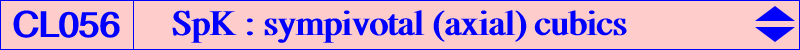Let P be a fixed point and let L be a line that is not the line at infinity. Let X be its infinite point and let X* be the isogonal conjugate of X. X* lies on the circumcircle (O) of ABC and Y* is the antipode of X* on (O). Thus Y is the infinite point of any perpendicular to L. Let (C) be the circumconic passing through the reflections A', B', C' of A, B, C about L. (C) meets (O) again at Z = XX* /\ YY*. Let l be a variable line through P and let l' be its reflection in L. The isogonal transform l* of l intersects l' at two points M, N. When l varies, the locus of M, N is a circumcubic we shall call the sympivotal (isogonal, axial) cubic SpK(P, L). Naturally, the isogonal transformation can be replaced by any other isoconjugation and the results below are easily adapted. See CL055 when the axial symmetry is replaced by a central symmetry and CL058 when it is replaced by a rotation.General properties of SpK(P, L) • SpK(P, L) is a circular circumcubic. • SpK(P, L) contains the reflection P' of P about L and the isogonal conjugate P* of P. • SpK(P, L) meets BC again at U = BC /\ P'A'. V and W are defined similarly. The points U' = AP* /\ UP', V' = BP* /\ VP' and W' = CP* /\ WP' also lie on SpK(P, L). • SpK(P, L) contains F1, F2 the two isogonal conjugate points symmetric about L. These points are not necessarily real nor distinct (when L contains an in/excenter of ABC). F1, F2 are the common points of three circles (Ca), (Cb), (Cc) defined as follows. The line AX* meets L at the center of (Ca) which passes through A. • SpK(P, L) meets L at three points L1, L2, L3 that lie on pK(X6, P). • The perpendicular L' to L at P meets SpK(P, L) at P' and two other isogonal conjugate points P1, P2 lying on the circumconic passing through P* and Y*. Obviously pK(X6, P) contains P1, P2 hence we know the nine common points of SpK(P, L) and pK(X6, P). • The isogonal transform of SpK(P, L) is SpK(P', L) and these two cubics are generally distinct i.e. SpK(P, L) is generally not a self-isogonal cubic. Their nine common points are A, B, C, F1, F2, P1, P2 and the circular points at infinity. • The parallel at P' to L meets SpK(P, L) at P' and two other points P3, P4 lying on the circumconic passing through P* and X*. • The third point F3 of SpK(P, L) on the line F1F2 (the radical axis of the three circles above) also lies on the line ZP'. This line meets (O) again at C6, the last common point of (O) and SpK(P, L). • The second intersection of (O) and ZP is the isogonal conjugate of the real infinite point of SpK(P, L). This latter point is also the infinite point of the line P*F3 hence the real asymptote of SpK(P, L) is parallel to the line P*F3. • SpK(P, L) is a K0 (without term in xyz) if and only if P lies on the perpendicular to L passing through the point Q0 which is the isogonal conjugate of the trilinear pole of L. If the equation of L is ux+vy+wz=0, this point Q0 is a^2u:b^2v:c^2w.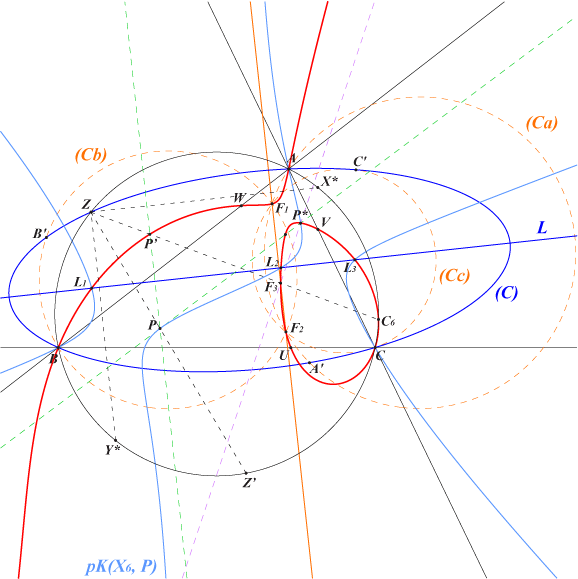SpK(P, L) with P on the line at infinity • When P = Y, the lines l and l' always coincide hence SpK(Y, L) is the isogonal circular pivotal cubic pK(X6, Y) with singular focus X*. The real asymptote is the line YY*. • When P = X, SpK(X, L) is the isogonal focal non-pivotal cubic nK(X6, R, X) with root R, the trilinear pole of the line passing through the (here) collinear points U, V, W. R is actually the complement of the isotomic conjugate of the trilinear pole of L. The singular focus is X* again. The real asymptote is the homothetic of L (the orthic line or axis of the cubic) under h(X*,2). This cubic is the locus of foci of inscribed conics centred on the Newton line of the quadrilateral formed with L and the sidelines of ABC. • When P is different of X and Y, we obtain two cubics SpK(P, L) and SpK(P', L) each being the isogonal transform of the other and both belonging to the pencil of circular cubics generated by the two cubics above. All the cubics of this pencil have the same singular focus namely X* and their asymptotes pass through a fixed point which is the intersection of the asymptotes of the two isogonal cubics above.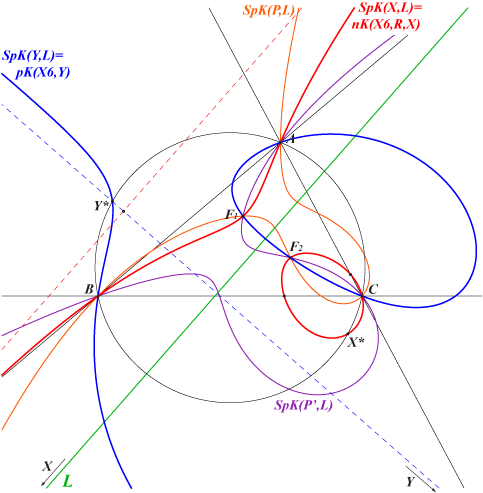One of the most interesting case is when L is the Brocard axis OK since F1, F2 are the Brocard points. We obtain a pencil of circular cubics generated by SpK(X512, OK) = pK(X6, X512) = K021 and SpK(X511, OK) = nK0(X6, X647) = K019. The common singular focus is the Tarry point X(98) and the asymptotes concur at X(99).SpK(P, L) with P on the line L When P lies on L, SpK(P, L) is an isogonal non-pivotal focal cubic passing through F1, F2, P and P*. It is the locus of foci of inscribed conics with center on the line passing through the midpoints of F1, F2 and P, P*. For a given line L, when P traverses L all these cubics form a pencil of focal cubics with circular focus on the circumcircle. For example, if we take the Euler line as line L, we obtain a pencil of isogonal focal nK passing through O and H. Each cubic is the locus of foci of inscribed conics with center on a line passing through the nine point center X(5). See K072, K164, K165, K166, K433 for instance.SpK(P, L) with collinear points U, V, W We already know that U, V, W are collinear when P lies on L since SpK(P, L) is a nK i.e. a non-pivotal isocubic. This also occurs when P lies on the circumconic that passes through the reflections A', B', C' of A, B, C about L. In this case, the line UVW is perpendicular to L and the cubic SpK(P, L) is a nK. Its root R lies on the circumconic with perspector Y and the isoconjugate of R lies on the trilinear polar of Y*. Its pole lies on a cK with singularity at X6.SpK(P, L) with cevian points U, V, W We already know that UVW is a cevian triangle when P = Y since SpK(Y, L) is a pK i.e. a pivotal cubic. More generally, SpK(P,L) meets the sidelines of ABC at the vertices U, V, W of a cevian triangle if and only if P lies on the axial pK with axis L. See CL057. It follows that, for a given line L, there are three points P such that SpK(P, L) is a pivotal cubic. These points are the intersections of the axial pK above with the perpendicular L0 at Q0 to L. One of them is Y giving the isogonal circular pK(X6, Y) already mentioned and the two (not always real) remaining points are symmetric about L. These give two pivotal cubics, each being the isogonal transform of the other. Two remarkable examples are given below when L is the orthic axis or the antiorthic axis.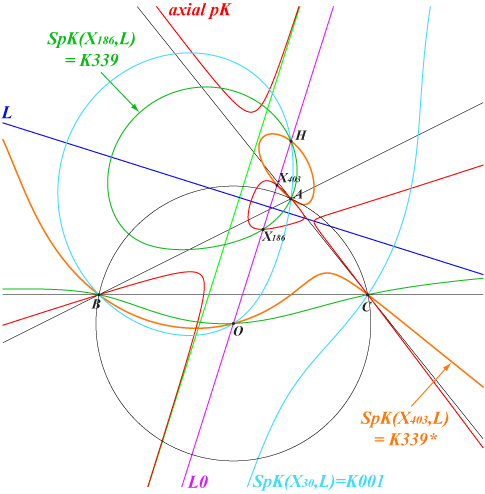L is the orthic axis L0 is the Euler line meeting the axial pK at X(30), X(186), X(403). SpK(X30, L) is the Neuberg cubic K001. SpK(X186, L) is K339 = pK(X3003, X4). SpK(X403, L) is the isogonal transform K339* of K339. The two points F1, F2 lie on the line X(30), X(50).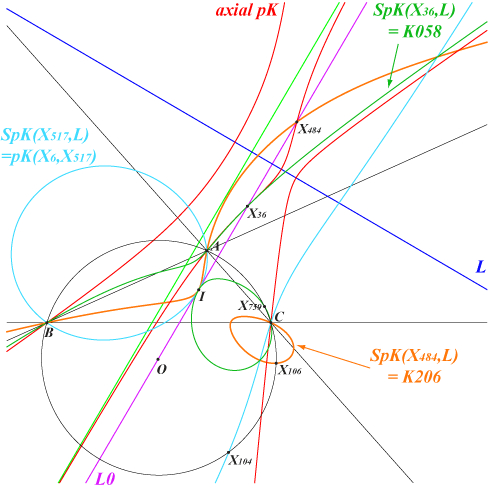L is the antiorthic axis L0 is the line OI meeting the axial pK at X(36), X(484), X(517). SpK(X517, L) is the circular pK(X6, X517). SpK(X36, L) is K058 = pK(X2161, X80). SpK(X484, L) is the isogonal transform K206 of K058. The two points F1, F2 lie on the line X(44), X(517).Special SpK(P, L) We study several interesting configurations for special positions of L with respect to point P.SpK(P on (O), PP*) is an axial isogonal focal nK We suppose that P is a point on the circumcircle (O) and that the line L is PP* i.e. the perpendicular at P to the Simson line of P. SpK(P, L) is an axial nK(X6, R, P) with root R on the line passing through the centroid G and the trilinear pole of the Simson line of P. P is the singular focus and the tangent at P is PP*. The axis of symmetry is the perpendicular at P to PP*. The circumconic isogonal transform of the line PP* meets PP* at two points E1, E2 which are the two other centers (apart P) of anallagmaty. The reflection of PP* about the midpoint of E1E2 is the real asymptote.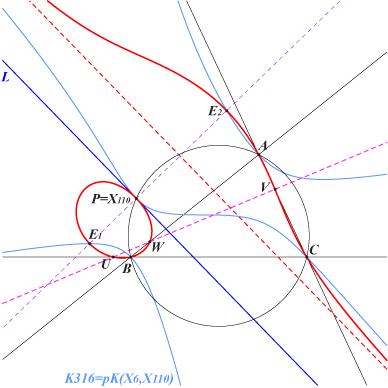The figure presents SpK(P, L) with P = X(110) – the focus of the Kiepert parabola and the singular focus of the Neuberg cubic K001). The line L is the perpendicular at X(110) to the Euler line. It is the axis of the Kiepert parabola. E1 and E2 are the common points of the parallel at X(110) to the Euler line and the circumconic passing through X(74) and X(523). These two points lie on K001 and on K316. The real asymptote is the perpendicular bisector of OH. See also CL027.SpK(P on L∞, PP*) is a central isogonal focal nK We suppose that P lies on the line at infinity hence P* is a point on (O). The line L = PP* is the perpendicular at P* to the Simson line S of P*.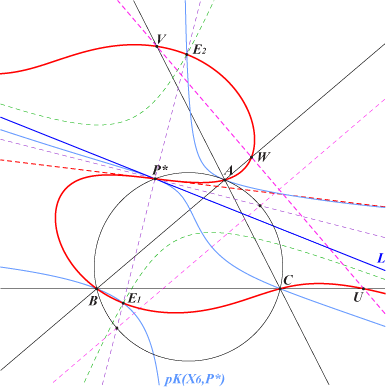SpK(P, L) is now a central isogonal focal nK with focus P*, the center of symmetry. The root R of the cubic is the homothetic of the trilinear pole of S under h(G, 1/4). The trilinear polar of R is obviously the line UVW. The perpendicular at O to UVW meets (O) at two points and the two lines passing through P* and these two points contain the two other real centers E1, E2 (apart P) of anallagmaty. E1, E2 are also the real foci of the inscribed conic with center P* hence they lie on the cubic pK(X6, P*). See CL001 and K084.SpK(P, PI) is a strophoid We suppose that P is not an in/excenter and we take L = PI, I being the incenter of ABC. In this case, SpK(P, L) is an isogonal strophoid with node I. See CL003.SpK(P, [PP*]) is a nodal cubic We suppose now that P is not an in/excenter and does not lie on (O) nor on the line at infinity. P and P* are distinct and finite points and the perpendicular bisector [PP*] of P and P* is defined. In such case, SpK(P, [PP*]) is a nodal cubic with node P*.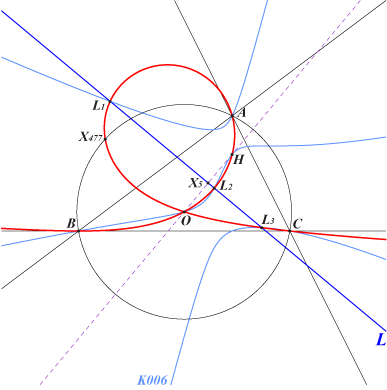The figure shows the case P = H, P* = O so that L is the perpendicular bisector of OH. SpK(H, [OH]) meets L at three points on the Orthocubic K006. The cubic has a node at O and it is not an isocubic.